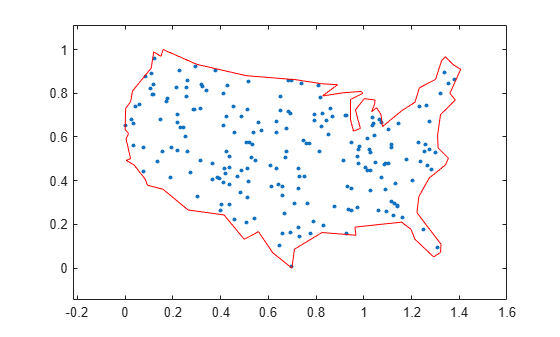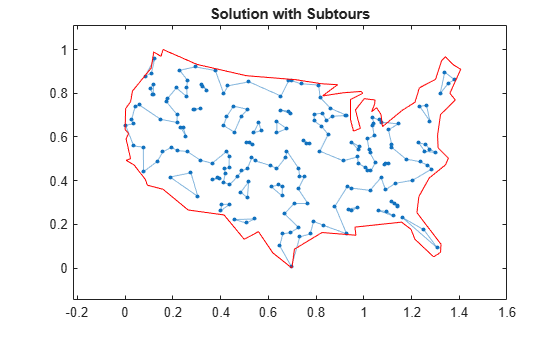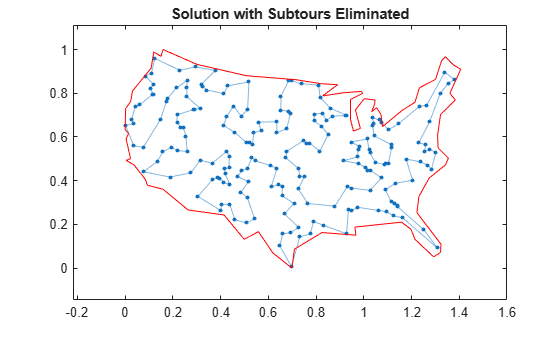# Traveling Salesman Problem: Problem-Based

This example shows how to use binary integer programming to solve the classic traveling salesman problem. This problem involves finding the shortest closed tour (path) through a set of stops (cities). In this case there are 200 stops, but you can easily change the `nStops` variable to get a different problem size. You'll solve the initial problem and see that the solution has subtours. This means the optimal solution found doesn't give one continuous path through all the points, but instead has several disconnected loops. You'll then use an iterative process of determining the subtours, adding constraints, and rerunning the optimization until the subtours are eliminated.

For the solver-based approach to this problem, see Traveling Salesman Problem: Solver-Based.

### Problem Formulation

Formulate the traveling salesman problem for integer linear programming as follows:

• Generate all possible trips, meaning all distinct pairs of stops.

• Calculate the distance for each trip.

• The cost function to minimize is the sum of the trip distances for each trip in the tour.

• The decision variables are binary, and associated with each trip, where each 1 represents a trip that exists on the tour, and each 0 represents a trip that is not on the tour.

• To ensure that the tour includes every stop, include the linear constraint that each stop is on exactly two trips. This means one arrival and one departure from the stop.

### Generate Stops

Generate random stops inside a crude polygonal representation of the continental U.S.

```load('usborder.mat','x','y','xx','yy'); rng(3,'twister') % Makes stops in Maine & Florida, and is reproducible nStops = 200; % You can use any number, but the problem size scales as N^2 stopsLon = zeros(nStops,1); % Allocate x-coordinates of nStops stopsLat = stopsLon; % Allocate y-coordinates n = 1; while (n <= nStops) xp = rand*1.5; yp = rand; if inpolygon(xp,yp,x,y) % tTest if inside the border stopsLon(n) = xp; stopsLat(n) = yp; n = n+1; end end```

### Calculate Distances Between Points

Because there are 200 stops, there are 19,900 trips, meaning 19,900 binary variables (# variables = 200 choose 2).

Generate all the trips, meaning all pairs of stops.

`idxs = nchoosek(1:nStops,2);`

Calculate all the trip distances, assuming that the earth is flat in order to use the Pythagorean rule.

```dist = hypot(stopsLat(idxs(:,1)) - stopsLat(idxs(:,2)), ... stopsLon(idxs(:,1)) - stopsLon(idxs(:,2))); lendist = length(dist);```

With this definition of the `dist` vector, the length of a tour is

`dist'*trips`

where `trips` is the binary vector representing the trips that the solution takes. This is the distance of a tour that you try to minimize.

### Create Graph and Draw Map

Represent the problem as a graph. Create a graph where the stops are nodes and the trips are edges.

`G = graph(idxs(:,1),idxs(:,2));`

Display the stops using a graph plot. Plot the nodes without the graph edges.

```figure hGraph = plot(G,'XData',stopsLon,'YData',stopsLat,'LineStyle','none','NodeLabel',{}); hold on % Draw the outside border plot(x,y,'r-') hold off```### Create Variables and Problem

Create an optimization problem with binary optimization variables representing the potential trips.

```tsp = optimproblem; trips = optimvar('trips',lendist,1,'Type','integer','LowerBound',0,'UpperBound',1);```

Include the objective function in the problem.

`tsp.Objective = dist'*trips;`

### Constraints

Create the linear constraints that each stop has two associated trips, because there must be a trip to each stop and a trip departing each stop.

Use the graph representation to identify all trips starting or ending at a stop by finding all edges connecting to that stop. For each stop, create the constraint that the sum of trips for that stop equals two.

```constr2trips = optimconstr(nStops,1); for stop = 1:nStops whichIdxs = outedges(G,stop); % Identify trips associated with the stop constr2trips(stop) = sum(trips(whichIdxs)) == 2; end tsp.Constraints.constr2trips = constr2trips;```

### Solve Initial Problem

The problem is ready to be solved. To suppress iterative output, turn off the default display.

```opts = optimoptions('intlinprog','Display','off'); tspsol = solve(tsp,'options',opts)```
```tspsol = struct with fields: trips: [19900×1 double] ```

### Visualize Solution

Create a new graph with the solution trips as edges. To do so, round the solution in case some values are not exactly integers, and convert the resulting values to `logical`.

```tspsol.trips = logical(round(tspsol.trips)); Gsol = graph(idxs(tspsol.trips,1),idxs(tspsol.trips,2),[],numnodes(G)); % Gsol = graph(idxs(tspsol.trips,1),idxs(tspsol.trips,2)); % Also works in most cases```

Overlay the new graph on the existing plot and highlight its edges.

```hold on highlight(hGraph,Gsol,'LineStyle','-') title('Solution with Subtours')```As can be seen on the map, the solution has several subtours. The constraints specified so far do not prevent these subtours from happening. In order to prevent any possible subtour from happening, you would need an incredibly large number of inequality constraints.

### Subtour Constraints

Because you can't add all of the subtour constraints, take an iterative approach. Detect the subtours in the current solution, then add inequality constraints to prevent those particular subtours from happening. By doing this, you find a suitable tour in a few iterations.

Eliminate subtours with inequality constraints. An example of how this works is if you have five points in a subtour, then you have five lines connecting those points to create the subtour. Eliminate this subtour by implementing an inequality constraint to say there must be less than or equal to four lines between these five points.

Even more, find all lines between these five points, and constrain the solution not to have more than four of these lines present. This is a correct constraint because if five or more of the lines existed in a solution, then the solution would have a subtour (a graph with $n$ nodes and $n$ edges always contains a cycle).

Detect the subtours by identifying the connected components in `Gsol`, the graph built with the edges in the current solution. `conncomp` returns a vector with the number of the subtour to which each edge belongs`.`

```tourIdxs = conncomp(Gsol); numtours = max(tourIdxs); % Number of subtours fprintf('# of subtours: %d\n',numtours);```
```# of subtours: 27 ```

Include the linear inequality constraints to eliminate subtours, and repeatedly call the solver, until just one subtour remains.

```% Index of added constraints for subtours k = 1; while numtours > 1 % Repeat until there is just one subtour % Add the subtour constraints for ii = 1:numtours inSubTour = (tourIdxs == ii); % Edges in current subtour a = all(inSubTour(idxs),2); % Complete graph indices with both ends in subtour constrname = "subtourconstr" + num2str(k); tsp.Constraints.(constrname) = sum(trips(a)) <= (nnz(inSubTour) - 1); k = k + 1; end % Try to optimize again [tspsol,fval,exitflag,output] = solve(tsp,'options',opts); tspsol.trips = logical(round(tspsol.trips)); Gsol = graph(idxs(tspsol.trips,1),idxs(tspsol.trips,2),[],numnodes(G)); % Gsol = graph(idxs(tspsol.trips,1),idxs(tspsol.trips,2)); % Also works in most cases % Plot new solution hGraph.LineStyle = 'none'; % Remove the previous highlighted path highlight(hGraph,Gsol,'LineStyle','-') drawnow % How many subtours this time? tourIdxs = conncomp(Gsol); numtours = max(tourIdxs); % Number of subtours fprintf('# of subtours: %d\n',numtours) end```
```# of subtours: 20 ```
```# of subtours: 7 ```
```# of subtours: 9 ```
```# of subtours: 9 ```
```# of subtours: 3 ```
```# of subtours: 2 ```
```# of subtours: 7 ```
```# of subtours: 2 ```
```# of subtours: 1 ```
```title('Solution with Subtours Eliminated'); hold off```### Solution Quality

The solution represents a feasible tour, because it is a single closed loop. But is it a minimal-cost tour? One way to find out is to examine the output structure.

`disp(output.absolutegap)`
``` 0 ```

The smallness of the absolute gap implies that the solution is either optimal or has a total length that is close to optimal.